# Balanced forcesPage 1

#### WATCH ALL SLIDES

Slide 1Balanced Forces

Slide 2## Levers

Slide 3## Write out the statements that are true.

a The longer the lever, the bigger the force that is needed to move an object.

b It is easier to close a door if you push the door close to the hinge

c The shorter the lever, the bigger the force that is needed to move an object

d Joints are examples of pivots.

e Bones are examples of levers.

Slide 4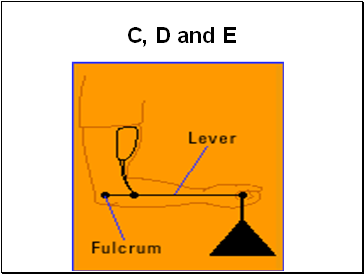C, D and E

Slide 5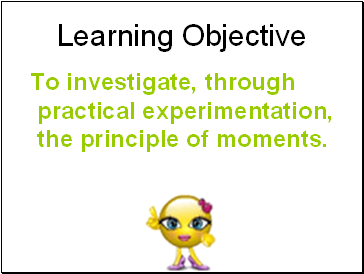## Learning Objective

To investigate, through practical experimentation, the principle of moments.

Slide 6Slide 7What do we need to record?

How many columns will we need in our table?

Slide 8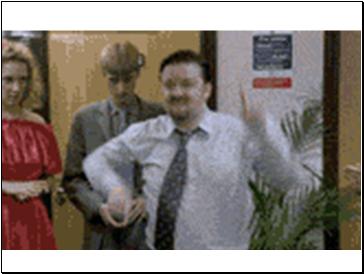Slide 9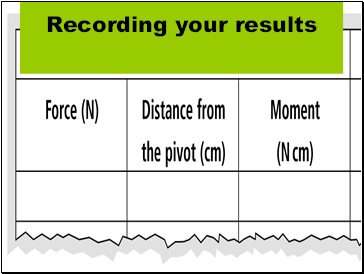Slide 10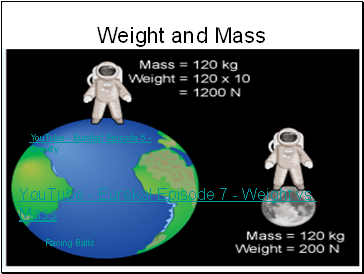Weight and Mass

YouTube - Eureka! Episode 7 - Weight vs. Mass

YouTube - Eureka! Episode 6 - Gravity

Racing Balls

Slide 11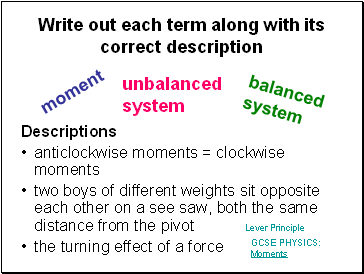## Write out each term along with its correct description

Descriptions

anticlockwise moments = clockwise moments

two boys of different weights sit opposite each other on a see saw, both the same distance from the pivot

the turning effect of a force

moment

balanced system

unbalanced system

Lever Principle

GCSE PHYSICS: Moments

Slide 12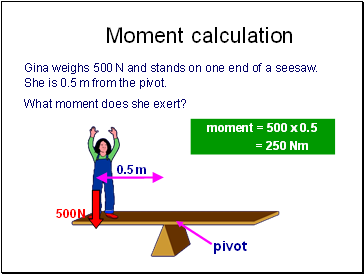## Moment calculation

Gina weighs 500 N and stands on one end of a seesaw. She is 0.5 m from the pivot.

What moment does she exert?

moment = 500 x 0.5

= 250 Nm

Slide 13## Moment equation

The moment of a force is given by the equation:

Moments are measured in Newton centimetres (Ncm) or Newton metres (Nm).

Slide 14## Principle of moments

The girl on the right exerts a clockwise moment, which equals .

Go to page:
1  2